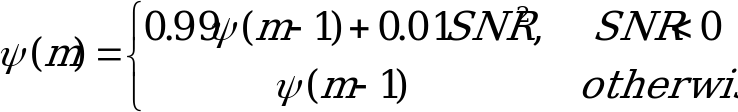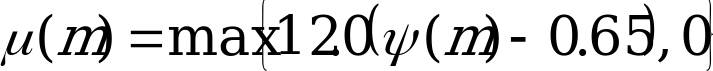## 4.3.6 Negative SNR Sensitivity Bias

06.943GPPTSVoice Activity Detector (VAD) for Adaptive Multi Rate (AMR) speech traffic channels

In order for the VAD decision to overcome the problem of being over-sensitive to fluctuating, non-stationary background noise conditions, a bias factor is used to increase the threshold on which the VAD decision is based. This bias factor is derived from an estimate of the variablility of the background noise estimate. The variability estimate is further based on negative values of the instantaneous SNR. It is presumed that a negative SNR can only occur as a result of fluctuating background noise, and not from the presence of voice. Therefore, the bias factor (m) is derived by first calculating the variability factor (m) as:(4.13)

which is then clamped in magnitude to. In addition, the variability factor is reset to zero when the frame count is less than or equal to four (m  4) or the forced update flag (sec 4.XX) is set (fupdate_flag == TRUE). The bias factor (m) is then calculated as:(4.14)